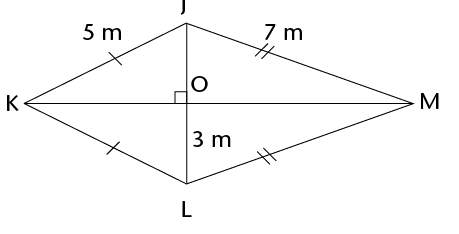In this chapter, you will revise how to calculate the perimeter and area of squares, rectangles, triangles and circles. The perimeter of a shape is the distance all the way around the sides of the shape. The area of a shape is the flat space inside the shape. You will also learn how to calculate the areas of parallelograms, rhombi, kites and trapeziums, as well as investigate the effect on the perimeter and area of a shape when its dimensions are doubled.

• Each block in figures A to F below measures 1 cm $\times$ 1 cm. What is the perimeter and area of each of the figures? Complete the table below.

The perimeter (P) of a shape is the distance along the sides of the shape. The area (A) of a figure is the size of the flat surface enclosed by the figure.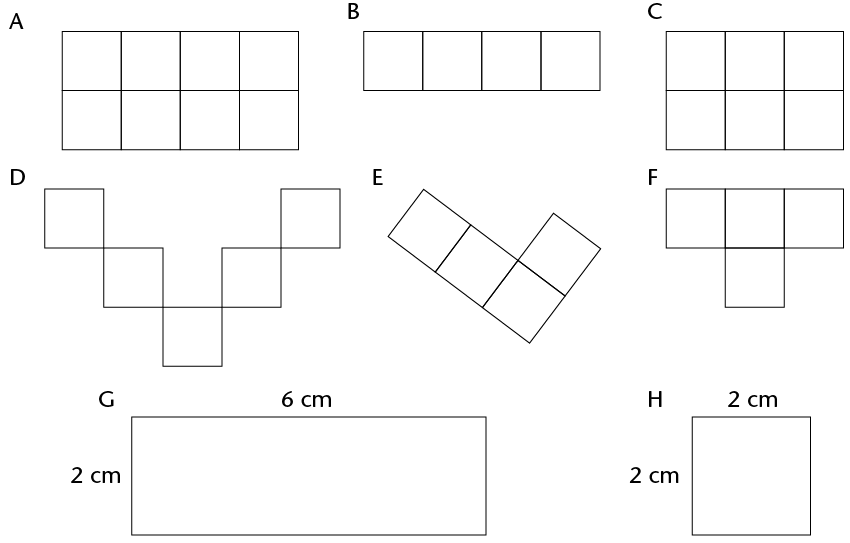Figure Perimeter Area Number of 1 cm $\times$ 1 cm squares A B C D E F G H
• Consider the rectangle below. It is formed by tessellating identical squares that are 1 cm by 1 cm each. The white part has squares that are hidden.

To tessellate means to cover a surface with identical shapes in such a way that there are no gaps or overlaps. Another word for tessellating is tiling.

1. Write down, without counting, the total number of squares that form this rectangle, including those that are hidden. Explain your reasoning.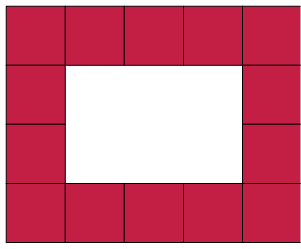2. What is the area of the rectangle, including the white part?

Area of a rectangle = length $\times$ breadth = $l \times b$

Area of a square = $l \times l = l^{2}$

Both length (l) and breadth (b) are expressed in the same unit.

• Sipho and Theunis each paint a wall to earn some money during the school holidays. Sipho paints a wall 4 m high and 10 m long. Theunis's wall is 5 m high and 8 m long. Who should be paid more? Explain.
• What is the area of a square with a length of 12 mm?
• The area of a rectangle is 72 cm2 and its length is 8 cm. What is its breadth?
• The diagram on the left below shows the floor plan of a room.
1. We can calculate the area of the room by dividing the floor into two rectangles, as shown in the diagram on the right below.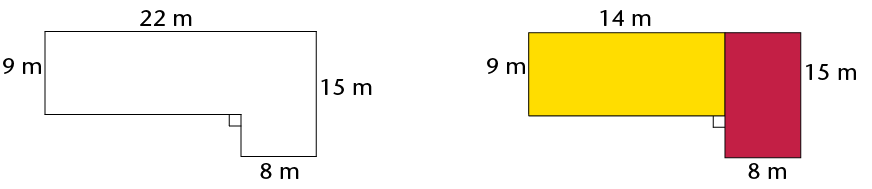Area of the room = Area of yellow rectangle + Area of red rectangle

$= (l \times b) + (l \times b)\\ = (14 \times 9) + (15 \times 8)\\ = 126 + 120\\ = 246 \text{ m}^{2}$

2. The yellow part of the room has a wooden floor and the red part is carpeted. What is the area of the wooden floor? What is the area of the carpet?
3. Calculate the area of the room using two different shapes. Draw a sketch.• Calculate the area of the figures below.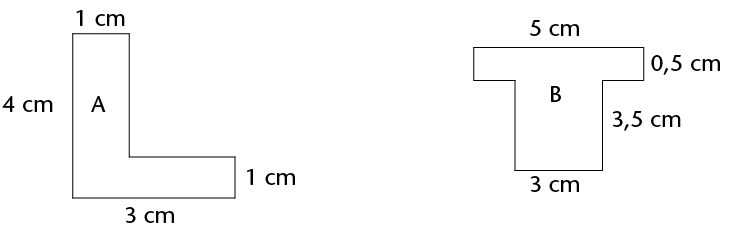• Which of the following rules can be used to calculate the perimeter (P) of a rectangle? Explain.
• Perimeter $= 2 \times (l + b)$
• Perimeter $= l + b + l + b$
• Perimeter $= 2l + 2b$
• Perimeter $= l + b$

l and b refer to the length and the breadth of a rectangle.

The following are equivalent expressions for perimeter:

$P = 2l + 2b$ and $P-2(l + b)$ and $P = l + b +l+b$

• Check with two classmates that the rule or rules you have chosen abover are correct ;then apply it to calculate the perimeter of figure A. Think carefully!
• The perimeter of a rectangle is 28 cm and its breadth is 6 cm. What is its length?
• circumference of a circle. You will remember the following aboutcircles from previous grades:
• The distance across the circle through its centre is called the diameter (d) of the circle.
• The distance from the centre of the circle to any point on the circumference is called the radius (r).
• The circumference (c) of a circle divided by its diameter is equal to the irrational value we call pi $(\pi)$. To simplify calculations, we often use the approximate values 3,14 or $\frac{22}{7}$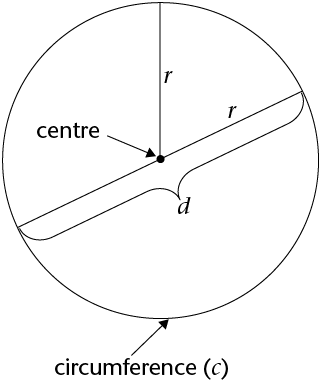• The following are important formulae to remember:

• $d = 2 \pi r$ and $r= \frac{1}{2}d$
• Circumference of a circle $(c) = 2 \pi r$
• Area of a circle $(A) = \pi r^{2}$
• Calculate the perimeter and area of the following circles:
1. A circle with a radius of 5 m
2. A circle with a diameter of 18 mm
• Calculate the radius of a circle with:
1. a circumference of 53 cm
2. a circumference of 206 mm
• Work out the area of the following shapes: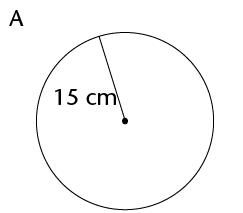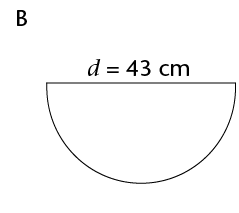• Calculate the radius and diameter of a circle with:
1. an area of 200 m2
2. an area of 1 000 m2
• Calculate the area of the shaded part.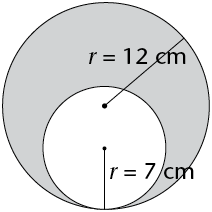• Remember:

1 cm = 10 mm 1 mm = 0,1 cm

1 m = 100 cm 1 cm = 0,01 m

1 km = 1 000 m 1 m = 0,001 km

• Convert the following:
1. 34 cm = .......... mm
2. 501 m = .......... km
3. 226 m = .......... cm
4. 0,58 km = .......... m
5. 1,9 cm = .......... mm
6. 73 mm = .......... cm
7. 924 mm = .......... m
8. 32,23 km = .......... m
• 2 to m2:
1 cm $\times$ 1 cm
=0.01 m $\times$ 0.01 m
=0.0001 m2 Example:
Convert 50 cm2to m2
1 cm2 = 0,0001 m2
50 cm2 = 50 $\times$ 0,0001 m2
= 0,005 m2
• Convert to cm2:
1. 650 mm2
2. 1 200 mm2
3. 18 m2
4. 0,045 m2
5. 93 mm2
6. 177 m2

1. Convert 93 mm2 to m2
2. Convert 0,017 km2 to m2
•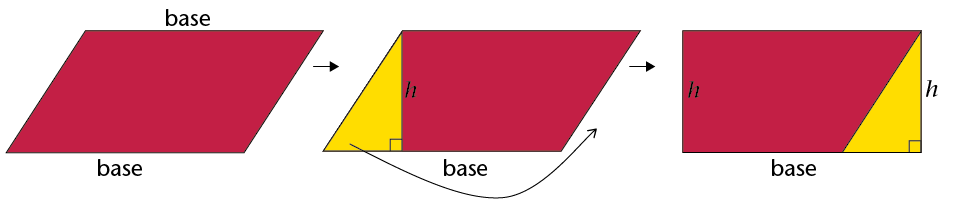$\therefore$ Area of parallelogram = base $\times$ perp. height

We can use any side of the parallelogram as the base, but we must use the perpendicular height on the side we have chosen.

1. Copy the parallelogram above into your exercise book.
2. Using the shorter side as the base of the parallelogram, follow the steps above to derive the formula for the area of a parallelogram.
• Work out the area of the following parallelograms using the formula.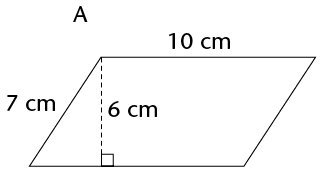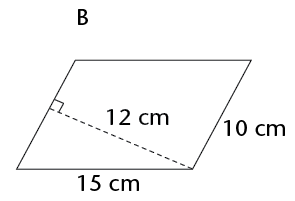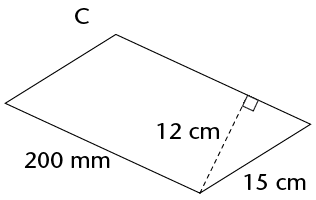• Work out the area of the parallelograms. Use the Theorem of Pythagoras to calculate the unknown sides you need. Remember to use the pre-rounded value for height and then round the final answer to two decimal places where necessary.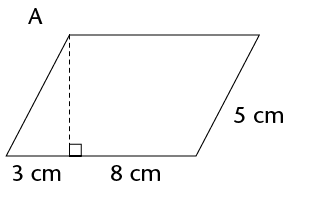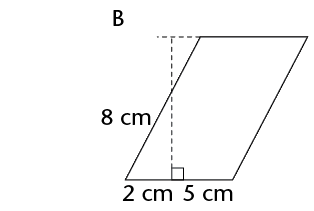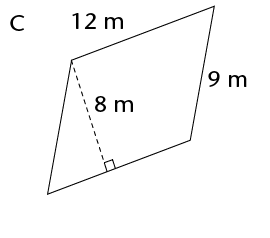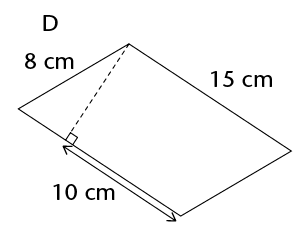• Area of a rhombus = length $\times$ perp. height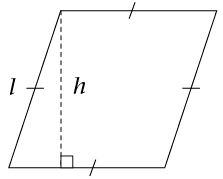• Work in your exercise book. Show how to derive the formula for the area of a rhombus.
• Calculate the areas of the following rhombi. Round off answers to two decimal places where necessary.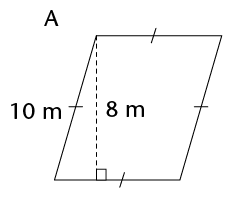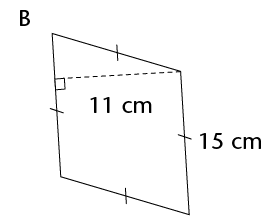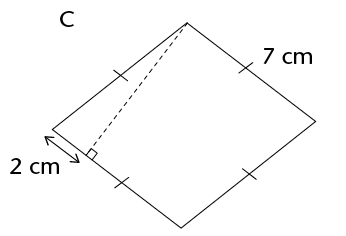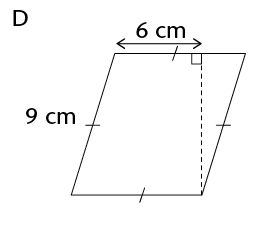• $\triangle$DEG + Area of $\triangle$EFG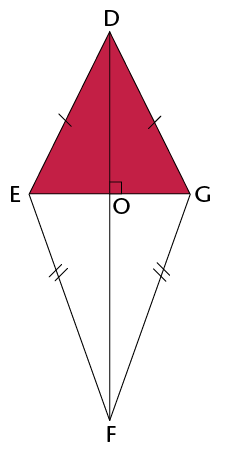$\therefore$ Area of a kite = $\frac{1}{2}$ (diagonal 1 $\times$ diagonal 2)

• Calculate the area of kites with the following diagonals. Give your answers in m2
1. 150 mm and 200 mm
2. 25 cm and 40 cm
• Calculate the area of the kite.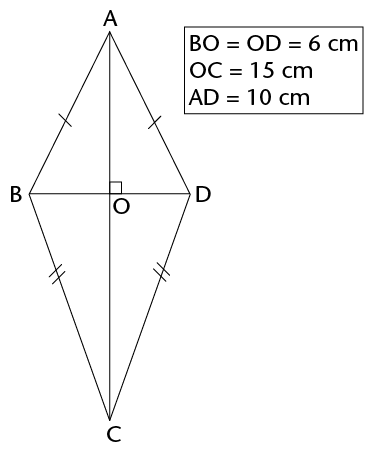•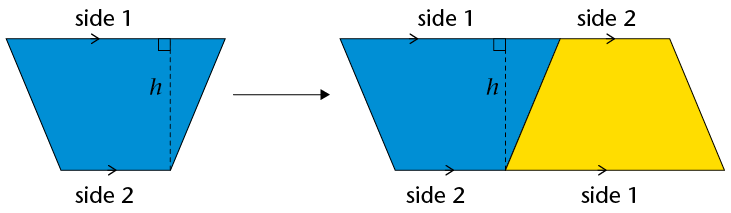$\therefore$ Area of a trapezium = $\frac{1}{2}$ (sum of parallel sides) $\times$ perp. height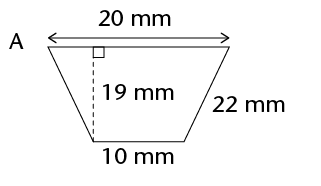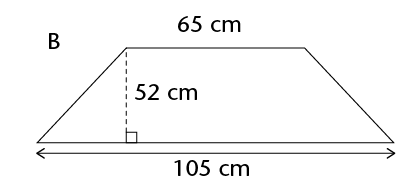• Calculate the areas of the following 2D shapes. Round off your answers to two decimal places where necessary.
1.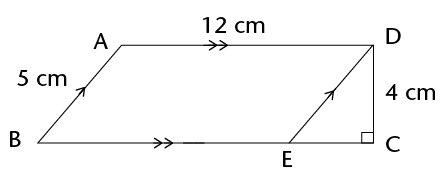2.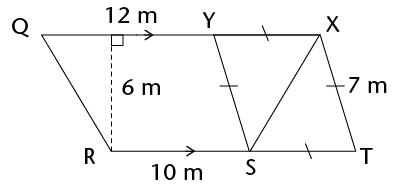3.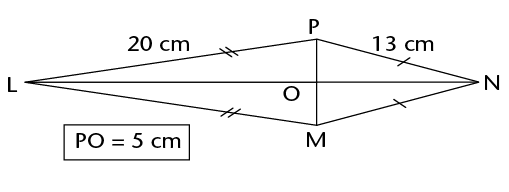4.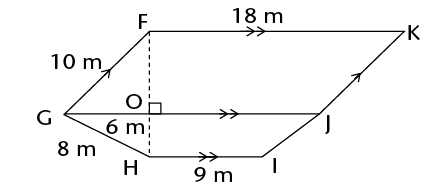• length and breadth for rectangles and squares
• bases and perpendicular heights for triangles, rhombi and parallelograms
• two diagonals for kites.
• Doubling means to multiply by 2.

• Work out the perimeter and area of each shape. Round off your answers to two decimal places where necessary.
• Which figure in each set is congruent to the original figure?
• Fill in the perimeter (P) and area (A) of each figure in the table below.
 Figure Original figure Figure with both dimensions doubled A P = A = P = A = B P = A = P = A = C P = A = P = A = D P = A = P = A =
• Look at the completed table above. What patterns do you notice? Choose one:
• When both dimensions of a shape are doubled, its perimeter is doubled and its area is doubled.
• When both dimensions of a shape are doubled, its perimeter is doubled and its area is four times bigger.
•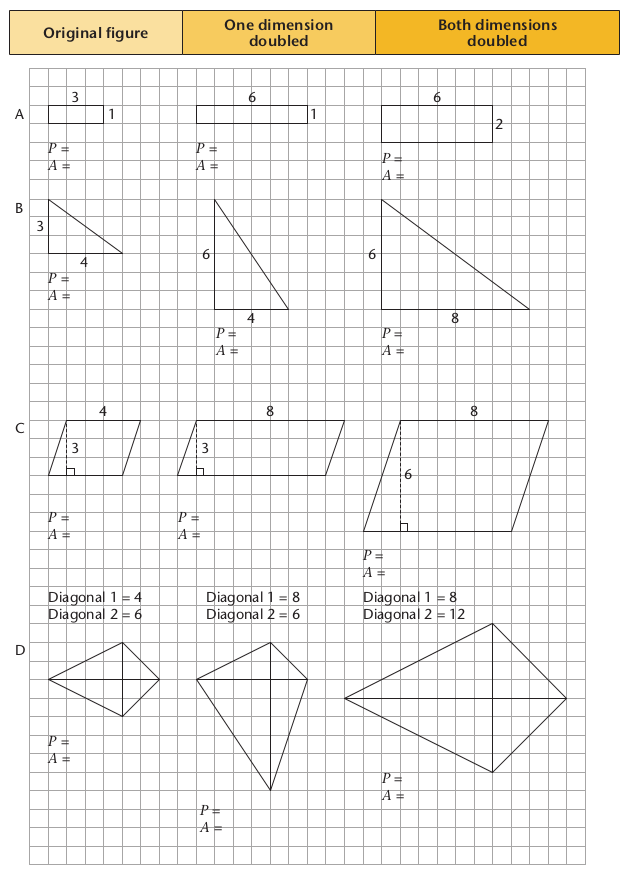1. Write down the formulae for the following:
 Perimeter of a square Perimeter of a rectangle Area of a square Area of a rectangle Area of a triangle Area of a rhombus Area of a kite Area of a parallelogram Area of a trapezium Diameter of a circle Circumference of a circle Area of a circle
1. Calculate the perimeter of the square and the area of the shaded parts of the square.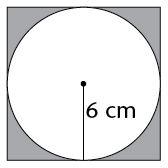2. Calculate the area of the kite.